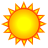# using multiprocessing in geoprocessing service

1046
6
02-18-2019 01:57 AMNew Contributor III

Hello everybody,

I have an arcpy program, that performs a series of different clips on an incoming geometry. I am only interested in the result, if there are geometries in the clip's result.

To use this tool in an web application, I have published it as a geoprocessing service,

For the sake of performance I want to calculate the several clips simultaneously.

I found this tutorial:

So I came to this solution (compressed to the basic I hope not have cut something important):

``````import time
import sys, os
from multiprocessing import Pool
import logging
import arcpy

logging.basicConfig(filename=os.path.join(arcpy.env.scratchWorkspace, "../", "20181204_BQ1_I.log"), level=logging.INFO, format='%(created)f %(asctime)s %(message)s')

# Some constants are set to link in the databases

out_path = "in_memory"
arcpy.env.workspace = out_path
arcpy.env.scratchWorkspace = out_path
out_name_geometry = "JustAName"

spatial_reference = arcpy.SpatialReference(2056)

def clipfun(result, kind, out_geometry,timeString,sde):
# the data will be set according to the kind
arcpy.analysis.Clip(kind   ,
arcpy.env.scratchGDB + "\\" + out_name_geometry + timeString,
out_geometry + "_Clip_" + kind)

rows = arcpy.da.SearchCursor(out_geometry + "_Clip_" + kind,
["SHAPE@AREA"])

result[kind+"_res"] = {}
ergebnis[kind+"_res"] = False
for row in rows:
if row >= schwellwertMeter:
result[kind+"_res"] = True
break
del rows
if row is not None:
del row
return result[kind+ "_res"]

if __name__ == '__main__':
geometry_as_json = arcpy.GetParameter(0)
# Set local variables
timeString = str(time.time()).replace(".", "_")
out_geometry = out_path + "\\" + out_name_geometry + timeString

# Execute CreateFeatureclass
arcpy.CreateFeatureclass_management(arcpy.env.scratchGDB,
out_name_geometry + timeString,
"POLYGON",
"#",
"DISABLED",
"DISABLED",
spatial_reference)
c = arcpy.da.InsertCursor(arcpy.env.scratchGDB + "\\" + out_name_geometry + timeString, ["SHAPE@JSON"])
c.insertRow([geometry_as_json])
del c

res={
"LV_res":"",
"NkB_res":"",
"NkBW_res": "",
"DG_res":""
ps = []
funcs=[(res,"LV",out_geometry,timeString,sde),
(res,"NkB",out_geometry,timeString,sde),
(res,"NkBW",out_geometry,timeString,sde),
(res,"DG",out_geometry,timeString,sde)]
p = Pool (processes=4)
erg = p.map(callfunction,funcs)

arcpy.SetParameter(1, res["LV_res"])
arcpy.SetParameter(2, res["NkB_res"])
arcpy.SetParameter(3, res["NkBW_res"])
arcpy.SetParameter(4, res["DG_res"])
logging.info('Ende')‍‍‍‍‍‍‍‍‍‍‍‍‍‍‍‍‍‍‍‍‍‍‍‍‍‍‍‍‍‍‍‍‍‍‍‍‍‍‍‍‍‍‍‍‍‍‍‍‍‍‍‍‍‍‍‍‍‍‍‍‍‍‍‍‍‍‍‍‍‍‍‍‍‍‍‍‍‍‍‍‍‍‍‍‍‍‍‍‍‍‍‍‍‍‍‍‍‍‍‍‍‍‍‍‍‍‍‍‍‍‍‍‍‍‍‍‍‍‍‍‍‍‍‍‍‍‍‍‍‍‍‍‍‍‍‍‍‍‍‍‍‍‍‍‍‍‍‍‍‍‍‍‍‍‍‍‍‍‍‍‍‍‍‍‍‍‍‍‍‍‍‍‍‍‍‍‍‍‍‍‍‍‍‍‍‍‍‍‍‍‍‍‍‍‍‍‍‍‍‍‍‍‍‍‍‍‍‍‍‍‍‍‍‍‍‍‍‍‍‍‍‍‍‍‍‍‍‍‍‍‍‍‍‍‍‍‍‍‍‍‍‍‍‍‍‍‍‍‍‍‍‍‍‍‍‍‍‍‍‍‍‍‍‍‍‍‍‍‍‍‍‍‍‍‍‍‍‍‍‍‍‍‍‍‍‍‍‍‍‍‍‍‍‍‍‍‍‍‍‍‍‍‍‍‍‍‍‍‍‍‍‍‍‍‍‍‍‍‍‍‍‍‍‍‍‍‍‍‍‍‍‍‍‍‍‍‍‍‍‍‍‍‍‍‍‍‍‍‍‍‍‍‍‍‍‍‍‍‍‍‍‍‍‍‍‍‍‍‍‍‍‍‍‍‍‍‍‍‍‍‍‍‍‍‍‍‍‍‍‍‍‍‍‍‍‍‍‍‍‍‍‍‍‍‍‍‍‍‍‍‍‍‍‍‍‍‍‍‍‍‍‍‍‍‍‍‍‍‍‍‍‍‍‍‍‍‍‍‍‍‍‍‍``````

I can run this Tool in an arcGIS for desktop Toolbox and I can publish it to an arcGIS for server as an geoprocessing service.

But when I try to run this new service I got this error:

Fehler bei der Ausführung des Werkzeuges. ITest Job ID: j0cc0f30e501247dba8e7877929cc45ec : Traceback (most recent call last): File "D:\arcgisserver\directories\arcgissystem\arcgisinput\I2.GPServer\extracted\v101\lokal\Inventare_bestaende_ITest.py", line 255, in erg = p.map(clipfun,funcs) File "C:\Python27\ArcGISx6410.5\Lib\multiprocessing\pool.py", line 251, in map return self.map_async(func, iterable, chunksize).get() File "C:\Python27\ArcGISx6410.5\Lib\multiprocessing\pool.py", line 567, in get raise self._value PicklingError: Can't pickle <type 'function'="">: attribute lookup __builtin__.function failed Fehler beim Ausführen von (I2). Fehler beim Ausführen von (ITest).

Has anybody done something like that and can help me with this?

Tank you,

Andreas Ruloffs

Tags (3)
6 RepliesbyMVP Legendary Contributor

Duncan Hornby had a post that may be of interest

are you using background geoprocessing?byMVP Esteemed ContributorNew Contributor III

Hallo,

thank you for your answers, but I don't see that they fit my problem.

The program works fine on the desktop but it fails after I have published it as a geoprocessing service on an arcgis for server. It seems to me, that the server has a different runtime environment than the desktop arcpy. I even don't if multiprocessing is possible on the server.

Tank you,

Andreas RuloffsbyMVP Esteemed Contributor

If you don't think that multiprocessing is possible on the server, why are you trying to run Python multiprocessing code on the server?

A vast majority of the time, Python error messages and trackbacks are specific and accurate.  Seeing the specific error is a PicklingError, and the module generating the error is multiprocessing, the SE thread I reference is directly related to your issue.New Contributor III

Dear Joshua,

I must perform a several independent clips on the incoming data and then generate an output like:

"The geometry interacts with: a water body, a national park and two woods"

So performing these clips via multiprocessing all together seems obvious to me.

Of cause you are right, that the SE thread matches my problem in a way, but I doen't see how I can influence this pickling.

Thank you,

Andreas RuloffsRegular Contributor III

From the looks of it, it seems you are just clipping 4 layers with a user defined polygon geometry and then inspecting the outputs... I think it would be much more efficient to use the Intersect tool to perform the goeprocessing here as opposed to 4, ideally simultaneous (but elusive) clips. Then you would scan the output using a searchcursor for the presence of the constituent records of the 4 inputs. Using the Intersect tool's join_attributes='ONLY_FID' parameter will probably make this more efficient if you just care about a yes/no presence. The right tool for the right job.